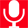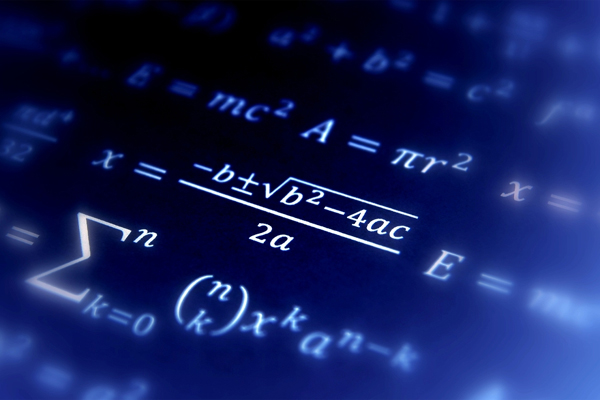Wednesday, December 6, 2023# Mathematics : IntroductionIntroduction Eligibility Job Prospects Institutes

Mathematics, one of the oldest and most fundamental sciences is the study of the measurement, properties and relationships of quantities and sets, using numbers and symbols. In simple words, mathematics deals with study of numbers and their various calculations. It is used throughout the world by all individuals in everyday life. Mathematics is an essential tool in many fields, including natural science, engineering, medicine and the social sciences. Mathematicians who deal in this subject field, use mathematical theory, computational techniques, algorithms, and the latest computer technology to solve economic, scientific, engineering, physics and business problems.

Mathematics is divided into two areas: theoretical or pure mathematics, and applied mathematics. Theoretical mathematics or pure mathematics is the study of the basic concepts and structures that underlie mathematics. Its purpose is to search for a deeper understanding and an expanded knowledge of mathematics itself. It is concerned with expanding and clarifying mathematical theories and laws. Applied mathematics is a branch of mathematics that concerns itself with mathematical methods that are typically used in science, engineering, business and industry. Thus, applied mathematics is a mathematical science with specialized knowledge.

Mathematics is used extensively in physics, actuarial science, statistics, engineering and operations research. Computer science, business and industrial management, economics, finance, chemistry, geology, life sciences, and behavioral sciences are likewise dependent on applied mathematics.

Tags
maths career

Find it Useful ? Help Others by Sharing Online